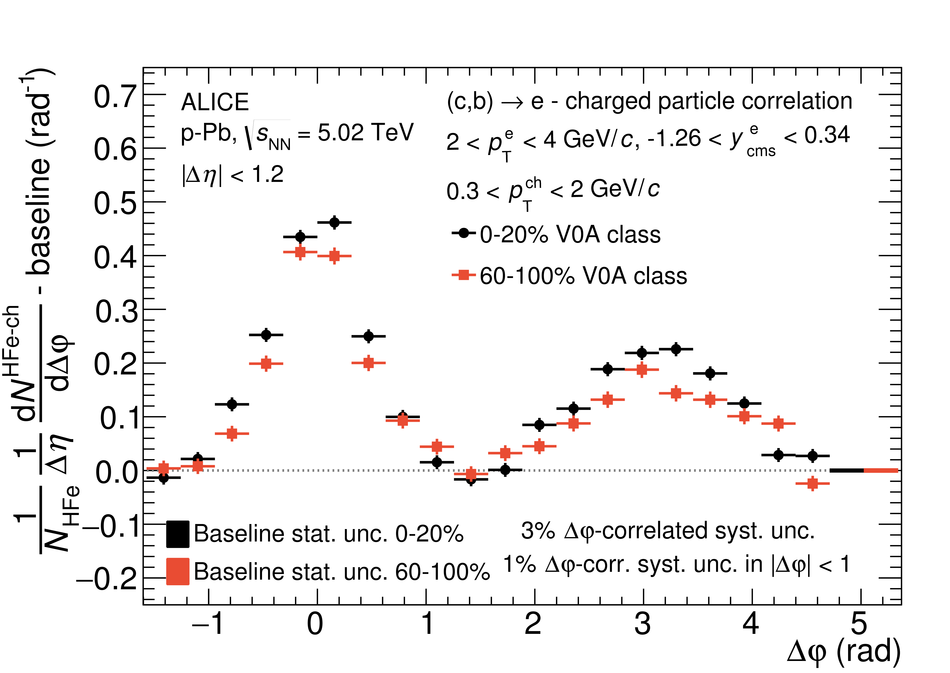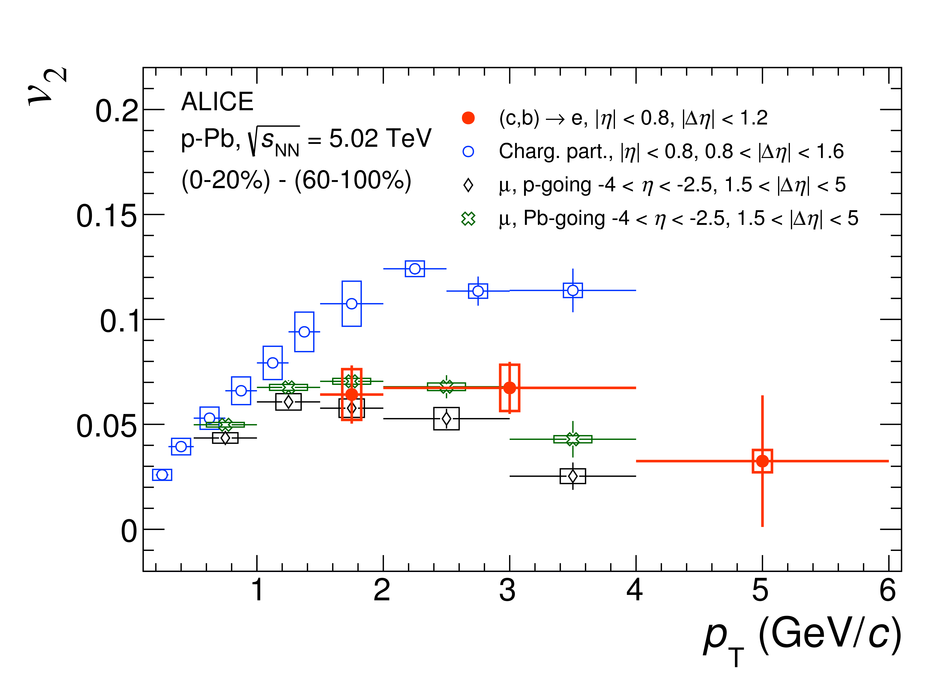# Azimuthal anisotropy of heavy-flavour decay electrons in p-Pb collisions at $\sqrt{s_{\rm NN}}$ = 5.02 TeV

Angular correlations between heavy-flavour decay electrons and charged particles at mid-rapidity ($|\eta| <~ 0.8$) are measured in p-Pb collisions at $\sqrt{s_{\rm NN}}$ = 5.02 TeV. The analysis is carried out for the 0-20% (high) and 60-100% (low) multiplicity ranges. The jet contribution in the correlation distribution from high-multiplicity events is removed by subtracting the distribution from low-multiplicity events. An azimuthal modulation remains after removing the jet contribution, similar to previous observations in two-particle angular correlation measurements for light-flavour hadrons. A Fourier decomposition of the modulation results in a positive second-order coefficient ($v_{2}$) for heavy-flavour decay electrons in the transverse momentum interval $1.5 <~ p_{\rm{T}} <~ 4$ GeV/$c$ in high-multiplicity events, with a significance larger than $5\sigma$. The results are compared with those of charged particles at mid-rapidity and of inclusive muons at forward rapidity. The $v_2$ measurement of open heavy-flavour particles at mid-rapidity in small collision systems could provide crucial information to help interpret the anisotropies observed in such systems.

Figures

## Figure 1

 Azimuthal correlations between heavy-flavour decay electrons and charged particles, for high-multiplicity and low-multiplicity p--Pb collisions, after subtracting the baseline (see text for details) for $2 < p_{\rm{T}}^{\rm e} < 4$ GeV/$c$ and $0.3 < p_{\rm T}^{\rm{ch}} < 2$ GeV/$c$.## Figure 2

 Best fit (Eq. \ref{Eq:fit}) to the azimuthal correlation distribution between heavy-flavour decay electrons and charged particles, for high-multiplicity p--Pb collisions after subtracting the jet contribution based on low-multiplicity collisions. The distribution is shown for $2 < p_{\rm{T}}^{\rm{e}} < 4$ GeV/$c$ and $0.3 < p_{\rm{T}}^{\rm{ch}} < 2$ GeV/$c$. The figure shows only statistical uncertainty## Figure 3

 Heavy-flavour decay electron $v_2$ as a function of transverse momentum compared to the $v_2$ of unidentified charged particles~ and inclusive muons~.